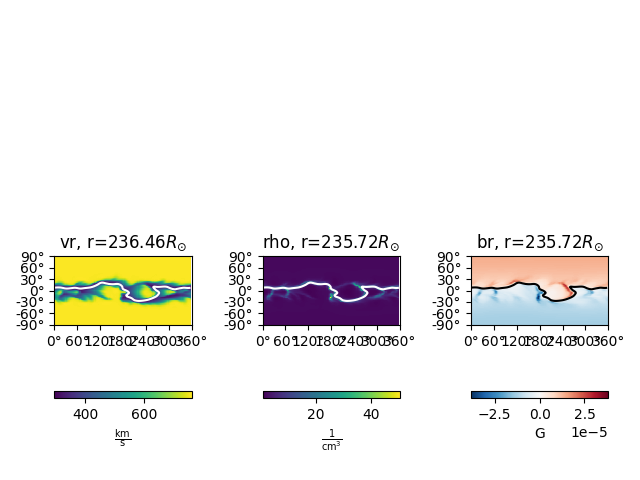This example shows how to plot slices of constant radial distance from a MAS model output.

```from psipy.model import MASOutput
from psipy.data import sample_data

import matplotlib.pyplot as plt
```

Next, load a set of MAS output files. You will need to change this line to point to a folder with MAS files in them.

```mas_path = sample_data.mas_helio()
model = MASOutput(mas_path)
```

Each MAS model contains a number of variables. The variable names can be accessed using the `.variables` attribute.

```print(model.variables)
```

Out:

```['br', 'rho', 'vr']
```

Set parameters for plotting. The first line will give us a horizontal errorbar underneath the plots. The second line is the index to select for the radial slice.

```cbar_kwargs = {'orientation': 'horizontal'}
r_idx = 139
```

Plot the slices

```fig, axs = plt.subplots(ncols=3, tight_layout=True)

ax = axs
ax = axs